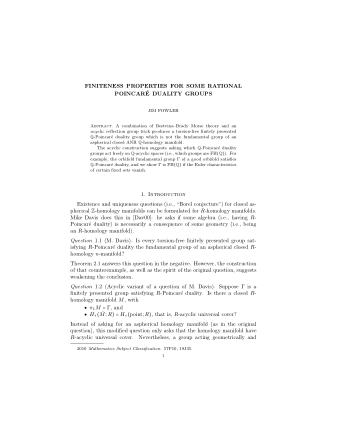Finiteness properties

A combination of Bestvina–Brady Morse theory and an acyclic reflection group trick produces a torsion-free finitely presented $\mathbb{Q}$-Poincaré duality group which is not the fundamental group of an aspherical closed ANR $\mathbb{Q}$-homology manifold.

The acyclic construction suggests asking which $\mathbb{Q}$-Poincaré duality groups act freely on $\mathbb{Q}$-acyclic spaces (i.e., which groups are $\mbox{FH}(\mathbb{Q})$). For example, the orbifold fundamental group $\Gamma$ of a good orbifold satisfies $\mathbb{Q}$-Poincaré duality, and we show $\Gamma$ is $\mbox{FH}(\mathbb{Q})$ if the Euler characteristics of certain fixed sets vanish.